It looks like you're using Internet Explorer 11 or older. This website works best with modern browsers such as the latest versions of Chrome, Firefox, Safari, and Edge. If you continue with this browser, you may see unexpected results.

# Math

Some of the content of this guide was modeled after a guide originally created by the Openstax and has been adapted for the GPRC Learning Commons in March 2021. The graphs are generated using Desmos. This work is licensed under a Creative Commons BY 4.0 International License.##### We start with the graph ofand shift the graph 4 units upward:##### We start with the original function which isand shift it 6 units to the right:3) f(cx), where c is a constant: c>1 compresses f(x) in the x direction and 0<c<1 stretches f(x).
##### The original function isand the function after transformation is. The constant number in the transformation iswhich means that it stretches the original function along the x axis.4) cf(x), where c is a constant: c>1 stretches the graph in the y direction and 0<c<1 compresses the graph.

##### The original function isand the constant coefficient iswhich stretches the graph in the y direction.5) -f(x): Reflects f(x) about the x axis.

##### Example: Sketch the graph of6) f(-x): Reflects f(x) about the y axis.

##### Example: Sketch the graph of##### The following graph shows the original function and all the transformations that results in the function given in this example:##### The original function and the transformations are shown in the diagram below:## Transformation using points on a graph

As the transformations are combined, a graph can be transformed point by point for simpler graphs.  For the transformation of the following form, the individual points of the graph can be transformed accordingly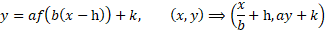In this example, take the graph above and apply the following transformations

*Equations will be replaced to match above at a later date*

• Transformation 1: Compression in the x-axis by a factor of 3
•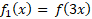• Transformation 2: Stretching in the y-axis by a factor of 2
•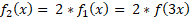• Transformation 3: Translation of 3 units to the right and 1 unit down
•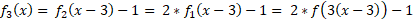This final form of the transformation written out in terms of initial x and y values to the transformed x and y values is as follows with the below graph showing the results of the transformation of each point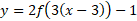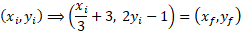​​​​​​​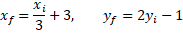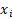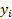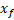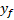-3

0

2

-1

0

3

3

5

3

-1

4

-3

6

2

5

3